# Non-Verbal Analogies - Logical Reasoning (MCQ) Questions

Dear Readers, Welcome to Logical Reasoning Non-Verbal Analogies questions and answers with explanation. These Non-Verbal Analogies solved examples with shortcuts and tricks will help you learn and practice for your Placement Test and competitive exams like Bank PO, IBPS PO, SBI PO, RRB PO, RBI Assistant, LIC,SSC, MBA - MAT, XAT, CAT, NMAT, UPSC, NET etc.

After practicing these tricky Non-Verbal Analogies multiple choice questions, you will be exam ready to deal with any objective type questions.

1)   The following question consists of some Problem Figures followed by other figures marked 1, 2, 3, 4 called the Answer Figures.

Find out the correct answer figure that should come next in the sequence of problem figures.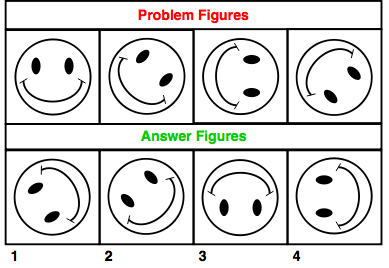- Published on 15 May 17

a. 1
b. 2
c. 3
d. 4
 Answer  Explanation ANSWER: 3 Explanation: The figure is rotated through 45 degrees in each step, i.e. 0, 45, 90, 135, 180 and so on.Thus, the answer figure should be figure 3.

2)   The following question consists of some Problem Figures followed by other figures marked 1, 2, 3, 4 called the Answer Figures.

Find out the correct answer figure that should come next in the sequence of problem figures.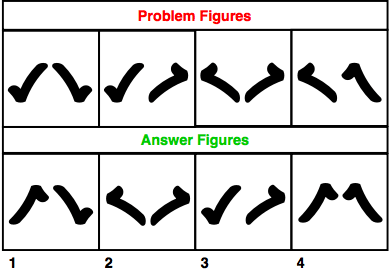- Published on 15 May 17

a. 1
b. 2
c. 3
d. 4
 Answer  Explanation ANSWER: 4 Explanation: The elements are mirror-images in the first step. In the next step, element on the right is rotated and in the next step, mirror-image is obtained. Similarly, in the next step, element on the right is rotated and the next figure should be the mirror-image. Thus, the answer figure should be figure 4.

3)   The following question consists of some Problem Figures followed by other figures marked 1, 2, 3, 4 called the Answer Figures.

Find out the correct answer figure that should come next in the sequence of problem figures.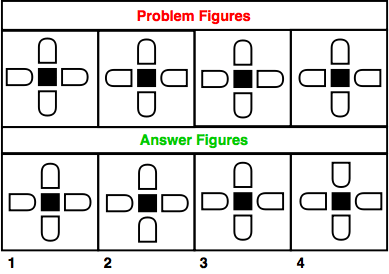- Published on 15 May 17

a. 1
b. 2
c. 3
d. 4
 Answer  Explanation ANSWER: 1 Explanation: The odd numbered figures are identical and the even numbered figures are identical. Thus, in the same order, the answer figure should be figure 1.

4)   The following question consists of some Problem Figures followed by other figures marked 1, 2, 3, 4 called the Answer Figures.

Find out the correct answer figure that should come next in the sequence of problem figures.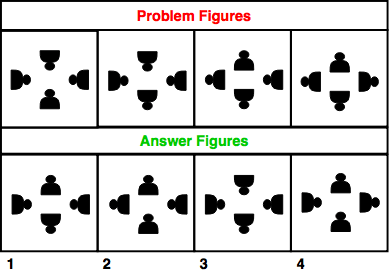- Published on 15 May 17

a. 1
b. 2
c. 3
d. 4
 Answer  Explanation ANSWER: 4 Explanation: The element at the bottom is rotated through 180 degrees.Then, two elements in the clockwise direction are rotated through 180 degrees.In the third step, the element to the right is rotated through 180 degrees.Thus, the next should be rotation of next two elements in the clockwise direction should be rotated by 180 degrees.The answer figure should be figure 4.

5)   The following question consists of some Problem Figures followed by other figures marked 1, 2, 3, 4 called the Answer Figures.

Find out the correct answer figure that should come next in the sequence of problem figures.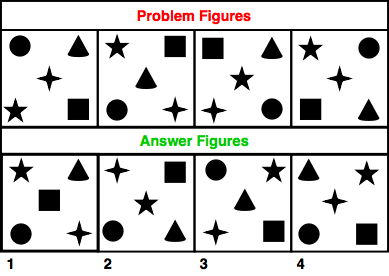- Published on 15 May 17

a. 1
b. 2
c. 3
d. 4
 Answer  Explanation ANSWER: 3 Explanation: The circle and square move in anti-clockwise direction in a set order.The elements in the centre follow a set order as - 4 - pointed star, triangle, 5-pointed star, 4-pointed star and so on.Thus, the answer figure should be figure 3.

6)   The following question consists of some Problem Figures followed by other figures marked 1, 2, 3, 4 called the Answer Figures.

Find out the correct answer figure that should come next in the sequence of problem figures.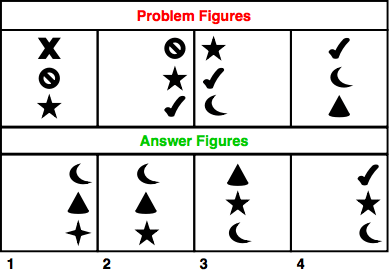- Published on 15 May 17

a. 1
b. 2
c. 3
d. 4
 Answer  Explanation ANSWER: 1 Explanation: 2nd element becomes the top element in the next figure. Third element becomes second element. The previous element is deleted and replaced with another.The complete column of figures moves one-third step forward each time. Thus, the answer figure should be figure 1.

7)   The following question consists of some Problem Figures followed by other figures marked 1, 2, 3, 4 called the Answer Figures.

Find out the correct answer figure that should come next in the sequence of problem figures.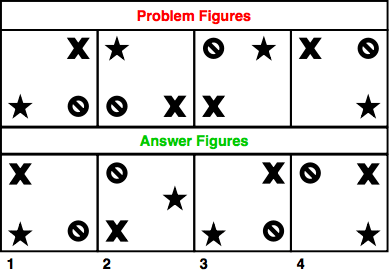- Published on 15 May 17

a. 1
b. 2
c. 3
d. 4
 Answer  Explanation ANSWER: 3 Explanation: The elements are moved forward in a clock-wise direction by single step each time. Thus, the answer figure should be figure 3.

8)   The following question consists of some Problem Figures followed by other figures marked 1, 2, 3, 4 called the Answer Figures.

Find out the correct answer figure that should come next in the sequence of problem figures.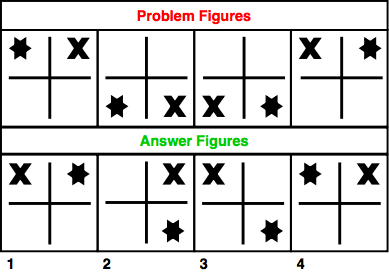- Published on 15 May 17

a. 1
b. 2
c. 3
d. 4
 Answer  Explanation ANSWER: 4 Explanation: X moves in clockwise direction and star moves in anti-clockwise direction in a set order. Thus, the answer figure should be figure 4.

9)   The following question consists of some Problem Figures followed by other figures marked 1, 2, 3, 4 called the Answer Figures.

Find out the correct answer figure that should come next in the sequence of problem figures.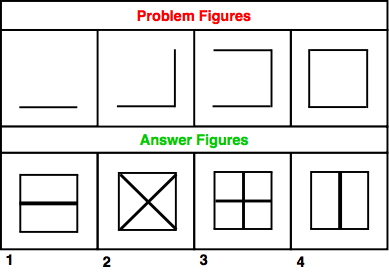- Published on 15 May 17

a. 1
b. 2
c. 3
d. 4
 Answer  Explanation ANSWER: 1 Explanation: Vertical and horizontal lines are added in each step to obtain a complete figure. Thus, the answer figure should be figure 1.

10)   The following question consists of some Problem Figures followed by other figures marked 1, 2, 3, 4 called the Answer Figures.

Find out the correct answer figure that should come next in the sequence of problem figures.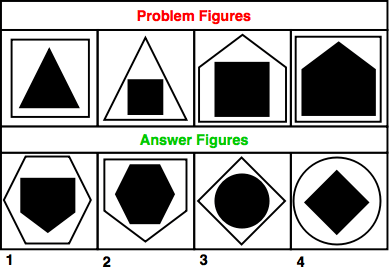- Published on 15 May 17

a. 1
b. 2
c. 3
d. 4
 Answer  Explanation ANSWER: 1 Explanation: 3-sided figure is inside 4-sided and in the next step it is reversed.Similarly, 4-sided figure is inside 5-sided figure and vice versa.Thus, following the same order, the answer figure should be figure 1.

1 2 3